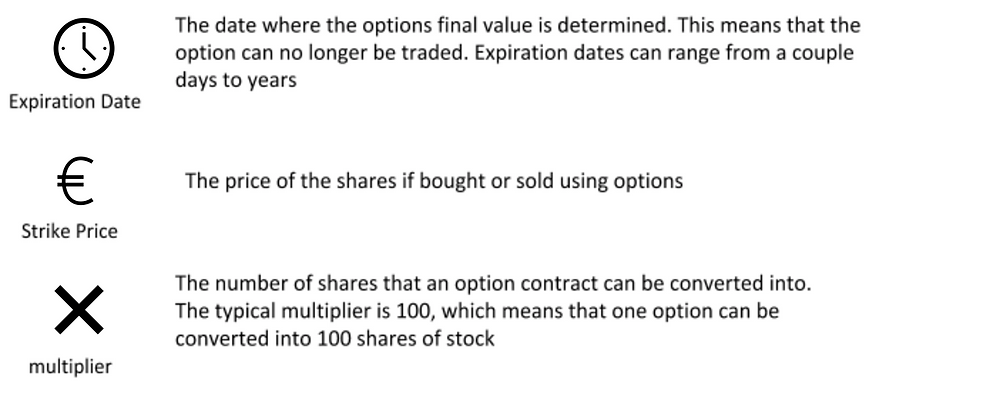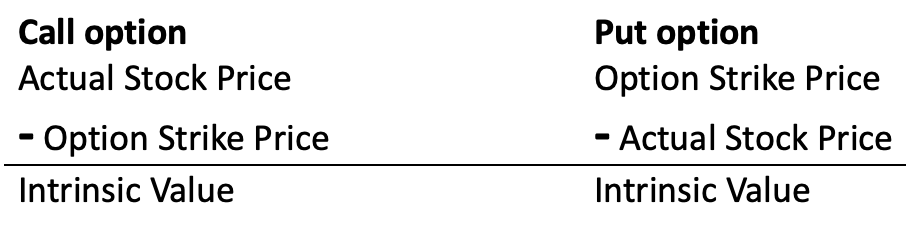Search
• Bocconi Students Options Club

# Calls, Puts and Option Pricing - The Basics

Although the idea of options may seem complex and confusing, it can actually become a very apprehendable concept once broken down. Therefore, we shall begin with the basics.

Now, unlike stocks, which are simply shares of firms that you may buy or sell, options are derivative contracts tied to an underlying asset (i.e. stocks). An option is a contract that gives you the right to sell or buy a certain asset (if you buy the contract) at a specific price (called the strike price). Options have a set of unique characteristics that are important to understand.It is quite easy to understand why a trader would opt for options rather than a simple stock trade, because long options can offer leveraged returns with minimal losses. The returns are leveraged as they offer you the same returns as if you owned 100 shares while only owning the contract. The only downside to options is that, while your profits may be immensely larger, your hypothetical loss would be of a larger scale too. Furthermore, you usually don’t own the underlying asset, therefore your contract can expire worthless rather easily. However, let us first analyze the 2 types of options: the call option and the put option.

## Call Options

A call option gives the buyer the right (but not the obligation) to buy 100 shares of stock per contract at the options strike price, before the option expires.

Here is a quick example to familiarize with calls.

Let us assume that there is a stock, priced at \$50, and that our prediction is that the stock will increase by \$10 after 60 days, therefore pushing the stock’s price to \$60. Say you buy the following call option: “strike:\$50 call expiring in 60 days”, and it is priced at \$5.00. Keep in mind, its price is multiplied by 100, as one contract represents 100 shares of the underlying. That a call with strike \$50 bought for \$5.00 will have a break-even point (BEP) at expiration of \$55 (found by adding the premium to the strike). Below is a chart showing your profits or losses from the call option, depending on what the underlying stock’s price movements after 60 days.## Put options

A put option gives the buyer the right (but not the obligation) to sell 100 shares of stock per contract at the options strike price, before the option expires. For simplicity, it is the opposite of a call option, since a profit is made through share prices decreasing. Here is a quick example to familiarize yourself with puts.

Let us assume that there is a stock, priced at \$200, and that our prediction is that the stock will decrease by \$10 within 30 days, therefore making the future stock price \$190. This time let’s assume you buy the following put: “strike:\$200 put expiring in 30 days”, and it is priced at \$6.50. Our break even point (BEP) will therefore be at 193.5 (200 - 6.50), where any movement of the stock below it will offer you profits. Below is a chart showing your profits or losses from the put option, depending on what the stock’s price would be at the 30 day mark.## Options Pricing

In order to know how much an option should be worth, you must firstly understand the two main components behind the creation of an option price: the intrinsic value and the extrinsic value. Once you combine these two values, you are able to determine the option’s price.

To begin, let us analyze the simpler value of the two, the intrinsic value.

• The intrinsic value is the “built-in” value of an option

• It is the difference between the strike price and the current underlying asset’s trading price.

• In the case of calls, the intrinsic value has a positive relationship with the stock price itself, meaning that if the stock increases, so does the intrinsic value.

• In the case of puts, they have a negative relationship, so if a stock goes up, the intrinsic value will decrease.

• It is not impacted by time of expiration

• The more intrinsic value, the more beneficial, since it is the only value which remains at the expiration date. If a stock has zero intrinsic value at expiration, it can be deemed worthless

For a more mathematical explanation, there are two formulas to determine the intrinsic value:For example:

If a stocks actual price was \$50, and the call option for that stock was at a strike of \$45, it would be more valuable to sell the stock at \$45 per stock then \$50, meaning that the option has a \$5 intrinsic “built-in” value (from the formula above).

However, if we were to take the example above, with the intrinsic value being \$5, the actual option price would still be higher than 5 euros due to an extra premium. That premium is in fact the second value which was mentioned above, the extrinsic value.

The extrinsic value has two subparts to it:

1. The time value. The more time an option has until its expiration date, the more time the underlying asset has to change. Thus, the more time the option has until expiration, the more valuable the option becomes. Therefore, the time value decreases over time and has no value at the expiration date.

2. Implied volatility. This effectively measures how much the stock price can vary over a specific set of time. It is represented as a percentage that shows the annualized expected one standard deviation range for the stock, based on the option price. For example, an implied volatility of 10% on a \$200 stock would represent a one standard deviation range of \$20 over the next year.

## How Options React to Price Change

There are multiple variables which may affect an options price, with different effects, so let us analyze the four main ones:

1. The strike price and underlying price. This may be said as the most straightforward and important variable of the four, as it is relatively obvious to say that call and put options are directly affected by changes in the price of their stock. As mentioned before, the value of an option increases when the stock increases (in a call option), and decreases when a stock goes up in value (for a put option), as it gives the holder of the option the right to buy or sell stocks at prices at a better rate than the market price.

2. Expiration date. The amount of time left until the expiration date of an option works in favor to the stock trader, and it is quite easy to conceptualize how this affects the price of an option. However, time to expiration works against a buyer since the value of an option may decline if there are no large changes in the price. Also, as seen before, the value of an option starts to significantly decline the closer it reaches its expiration date. On the other hand, time decay may benefit a seller of an option since the price declines.

3. Volatility. The more volatile an underlying asset is, the more expensive its options will be. Therefore, if an asset’s volatility greatly increases or decreases the price of options will be impacted (look up IV crush for example). Volatility may be one of the harder concepts in option pricing, as it relies on a statistical measure of volatility (SV), which observes previous price movements of the stock over a period of time. It may be more difficult to understand, because it requires a sort of educated guess on the price changes, using previous data. In a sense, a trader may have to work “backwards” while using the pricing model, by viewing different aspects, such as dividends, time, and interest rates. By using this data, you may find the key measure, called implied volatility (IV), for evaluating future prices.

See All